浙江大学 985 双一流 211

Zhejiang University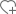关注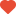已关注•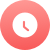1897

•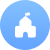公立

•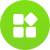综合

•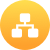教育部

•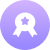本科

•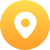浙江-杭州市

•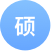398个

•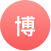332个

院校分数线

多方向招生特色专业

• 新闻学
• 软件工程(设3个专业方向)
• 信息与通信工程
• 动物科学
• 农林经济管理
• 电子信息工程
• 数字媒体技术
• 农业资源与环境
• 临床医学
• 工业设计
• 生物医学工程
• 建筑学
• 高分子材料与工程
• 过程装备与控制工程
• 能源与环境系统工程
• 环境科学
• 汉语言文学
• 数学与应用数学
• 机械电子工程
• 化学工程与工艺
• 生物科学
• 自动化
• 教育学
• 物理学
• 机械工程及自动化
• 信息工程
• 电气工程及其自动化
• 环境工程
• 国际经济与贸易
• 土木工程
• 植物保护
• 计算机科学与技术
• 生物系统工程
• 材料科学与工程
• 电子科学与技术院系/专业

• 英语（本）
• 法语（本）
• 俄语（本）
• 翻译（本）
• 日语（本）
• 德语（本）

• 历史学（本）
• 古典文献学（本）
• 编辑出版学（本）
• 哲学（本）
• 汉语言文学（本）
• 文物与博物馆学（本）

• 财政学（本）
• 国际经济与贸易（本）
• 经济学（本）
• 金融学（本）

• 法学（本）

• 教育学（本）
• 公共事业管理（本）
• 体育教育（本）
• 武术与民族传统体育（本）
• 运动训练学（本）
• 体育经济与管理（本）

• 地理信息科学（本）
• 地质学（本）
• 人文地理与城乡规划（本）
• 大气科学（本）
• 地球信息科学与技术（本）

• 市场营销（本）
• 财务管理（本）
• 人力资源管理（本）
• 信息管理与信息系统（本）
• 物流管理（本）
• 旅游管理（本）
• 会计学（本）

• 化学（本）
• 应用化学（本）

• 数学与应用数学（本）
• 统计学（本）
• 信息与计算科学（本）

• 应用心理学（本）

• 行政管理（本）
• 国际政治（本）
• 政治学与行政学（本）
• 社会学（本）
• 信息资源管理（本）
• 农林经济管理（本）

• 物理学（本）

• 光电信息科学与工程（本）

• 材料科学与工程学（本）

• 生物工程（本）
• 制药工程（本）
• 化学工程与工艺（本）

• 能源与环境统工程(热能方向和低温方向)（本）
• 新能源科学与工程（本）
• 过程装备与控制工程（本）
• 机械设计制造及其自动化(汽车工程)（本）

• 机械电子工程（本）
• 机械工程及自动化（本）
• 工业工程（本）

• 自动化（本）

• 海洋工程与技术（本）
• 港口航道与海岸工程（本）
• 船舶与海洋工程（本）
• 海洋科学（本）

• 生物信息学（本）
• 生物科学（本）
• 生态学（本）
• 生物技术（本）

• 高分子材料与工程（本）

• 测控技术与仪器（本）
• 生物医学工程（本）

• 微电子科学与工程（本）
• 电子科学与技术（本）
• 信息工程（本）

• 工业设计（本）
• 计算机科学与技术（本）
• 软件工程（本）
• 数字媒体技术（本）

• 生物系统工程（本）
• 食品科学与工程（本）

• 自动化（本）
• 电气工程及其自动化（本）
• 电子信息工程（本）

• 茶学（本）
• 农学（本）
• 园艺（本）
• 植物保护（本）
• 应用生物科学（本）
• 园林（本）

• 资源环境科学（本）
• 农业资源与环境（本）
• 环境科学（本）
• 环境工程（本）

• 预防医学(公共卫生)（本）
• 口腔医学（本）
• 基础医学（本）
• 临床医学（本）

• 交通工程（本）
• 建筑学（本）
• 土木工程（本）
• 城市规划（本）
• 水资源与海洋工程(水利工程)（本）

• 药物制剂（本）
• 药学（本）

• 飞行器设计与工程（本）
• 工程力学（本）

• 动物科技（本）
• 动物医学（本）

大学排名知名校友

•李彦宏

•俞洪敏

•撒贝宁

•马云400-181-5008

(免长话费 工作日 09:00-17:30)